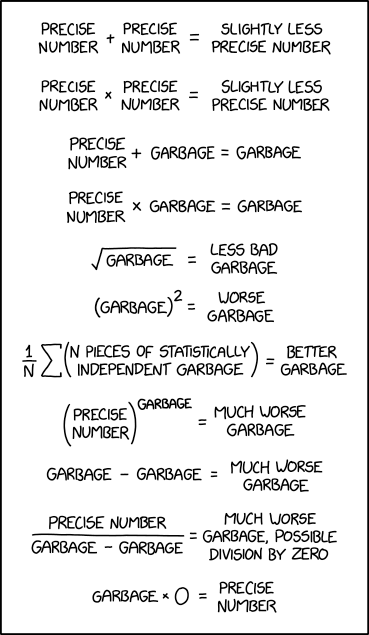# Garbage math

I really liked xkcd’s take on numerical analysis and error propagation:Source: https://xkcd.com/2295/

A good mathematical explanation of this comic can be found here: https://www.explainxkcd.com/wiki/index.php/2295:_Garbage_Math

# Fun with dimensional analysisSource: https://xkcd.com/2327/

# Chemistry Puns# Periodic# Mirror Image Symmetry from Different Viewpoints

Erica Flapan (Pomona College) explains why it is important to determine whether a molecule has mirror image symmetry, and discusses the differences between a geometric, chemical, and topological approach to understanding mirror image symmetry.

# Exponential growth and decay (Part 11): Half-life

In this series of posts, I provide a deeper look at common applications of exponential functions that arise in an Algebra II or Precalculus class. In the previous posts in this series, I considered financial applications. In today’s post, I’ll discuss radioactive decay and the half-life formula.

One way of writing the formula for how a radioactive substance (carbon-14, uranium-235, brain cells) decays is$A(t) = A_0 e^{-kt}$

In yesterday’s post, I showed how this formula is a natural consequence of a certain differential equation. Of course, students in Algebra II or Precalculus (or high school chemistry) are usually not ready to understand this derivation using calculus. Instead, they are typically given the final formula and are expected to use this formula to solve problems. Still, I think it’s important for the teacher of Algebra II or Precalculus to be aware of how the origins of this formula, as it only requires mathematics that’s only a year or two away in these students’ mathematical development.

There is another correct way to write this formula in terms of half-life. Sadly, my experience is that many students are familiar with these two different formulas but are not aware of how the two formulas are connected. As we’ll see, while yesterday’s post using differential equations is inaccessible to Algebra II and Precalculus students, the derivation below is entirely elementary and can be understood by good students in these courses.

Let$h$ be the half-life of the substance. By definition, this means that$A(h) = \displaystyle \frac{1}{2} A_0$

Substituting into the above formula, we find$\displaystyle \frac{1}{2} A_0 = A_0 e^{-kh}$

Let’s now solve for the constant$k$ in terms of$h$:$\displaystyle \frac{1}{2} = e^{-kh}$$\displaystyle \ln \left( \frac{1}{2} \right) = - k h$$\displaystyle -\frac{1}{h} \ln \left( \frac{1}{2} \right) = k$

Let’s now substitute this back into the original formula:$A = A_0 e^{-kt}$$A = A_0 e^{ -\left[ -\frac{1}{h} \ln \left( \frac{1}{2} \right) \right] t}$$A = A_0 e^{\ln \left( \frac{1}{2} \right) \cdot \frac{t}{h}}$$A = A_0 \left[e^{\ln \left( \frac{1}{2} \right)} \right]^{t/h}$$A = A_0 \displaystyle \left( \frac{1}{2} \right)^{t/h}$

This is the usual half-life formula, where the previous base of$e$ has been replaced by a new base of$\displaystyle \frac{1}{2}$ . For most applications, a base of$e$ is preferred. However, for historical reasons, the base of$\displaystyle \frac{1}{2}$ is preferred for problems involving radioactive decay. For example,$A(2h) = A_0 \displaystyle \left( \frac{1}{2} \right)^{2h/h}$$A(2h) = A_0 \displaystyle \left( \frac{1}{2} \right)^{2}$$A(2h) = \displaystyle \frac{1}{4} A_0$

In other words, after two half-life periods, only one-fourth (half of a half) of the substance remains.

# Exponential growth and decay (Part 10): Half-life

In this series of posts, I provide a deeper look at common applications of exponential functions that arise in an Algebra II or Precalculus class. In the previous posts in this series, I considered financial applications. In today’s post, I’ll discuss radioactive decay and the half-life formula.

While these formulas are easy to state, not many high school teachers are aware of the physical principles from which they arise. The basic idea is that if amount$A$ of a radioactive substance (carbon-14, uranium-235, brain cells) is present, the rate at which the substance decays is proportional to the amount of the substance currently present. This can be rewritten as a differential equation, since the rate at which the substance decays is$dA/dt$. So we find that$\displaystyle \frac{dA}{dt} = - k A$

The negative sign on the right-hand side isn’t strictly necessary, but it’s a reminder that amount present decreases as time increases.

This differential equation can be solved in several ways, including separation of variables (below, I’ll be sloppy with the constant of integration for the sake of simplicity):$\displaystyle \frac{dA}{A} = -k$$\displaystyle \int \frac{dA}{A} = - \displaystyle \int k \, dt$$ln |A| = -k t + C$$|A| = e^{-kt+C}$$|A| = e^{-kt} e^C$$A = \pm e^C e^{-kt}$$A = C e^{-kt}$

To solve for the constant, we usually use the initial condition$A(0) = A_0$, a number that must be given in the problem:$A(0) = C e^{-k \cdot 0}$$A_0 = C \cdot 1$$A_0 = C$

Plugging back in, we obtain the final answer$A(t) = A_0 e^{-kt}$

Of course, students in Algebra II or Precalculus (or high school chemistry) are usually not ready to understand this derivation using calculus. Instead, they are typically given the final formula and are expected to use this formula to solve problems. Still, I think it’s important for the teacher of Algebra II or Precalculus to be aware of how the origins of this formula, as it only requires mathematics that’s only a year or two away in these students’ mathematical development.

# Interdisciplinary studies (Part 4)

Shamelessly stolen from a friend:

How do you tell the difference between a plumber and a chemist?